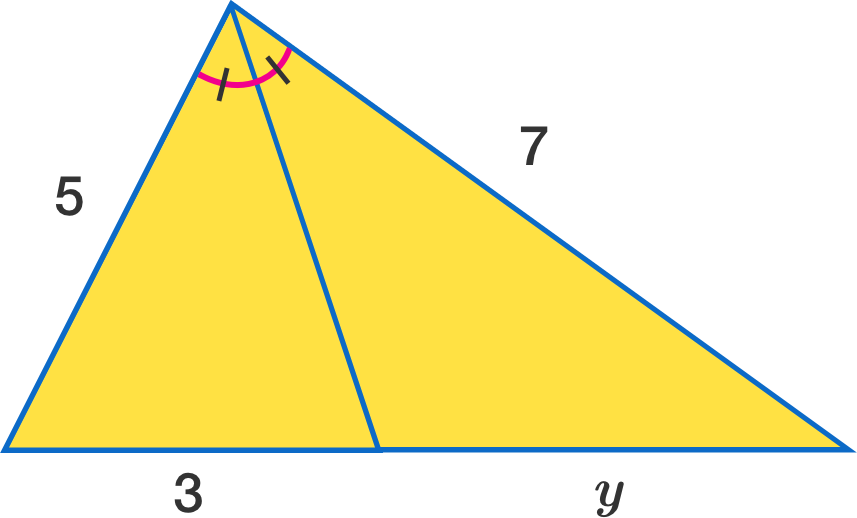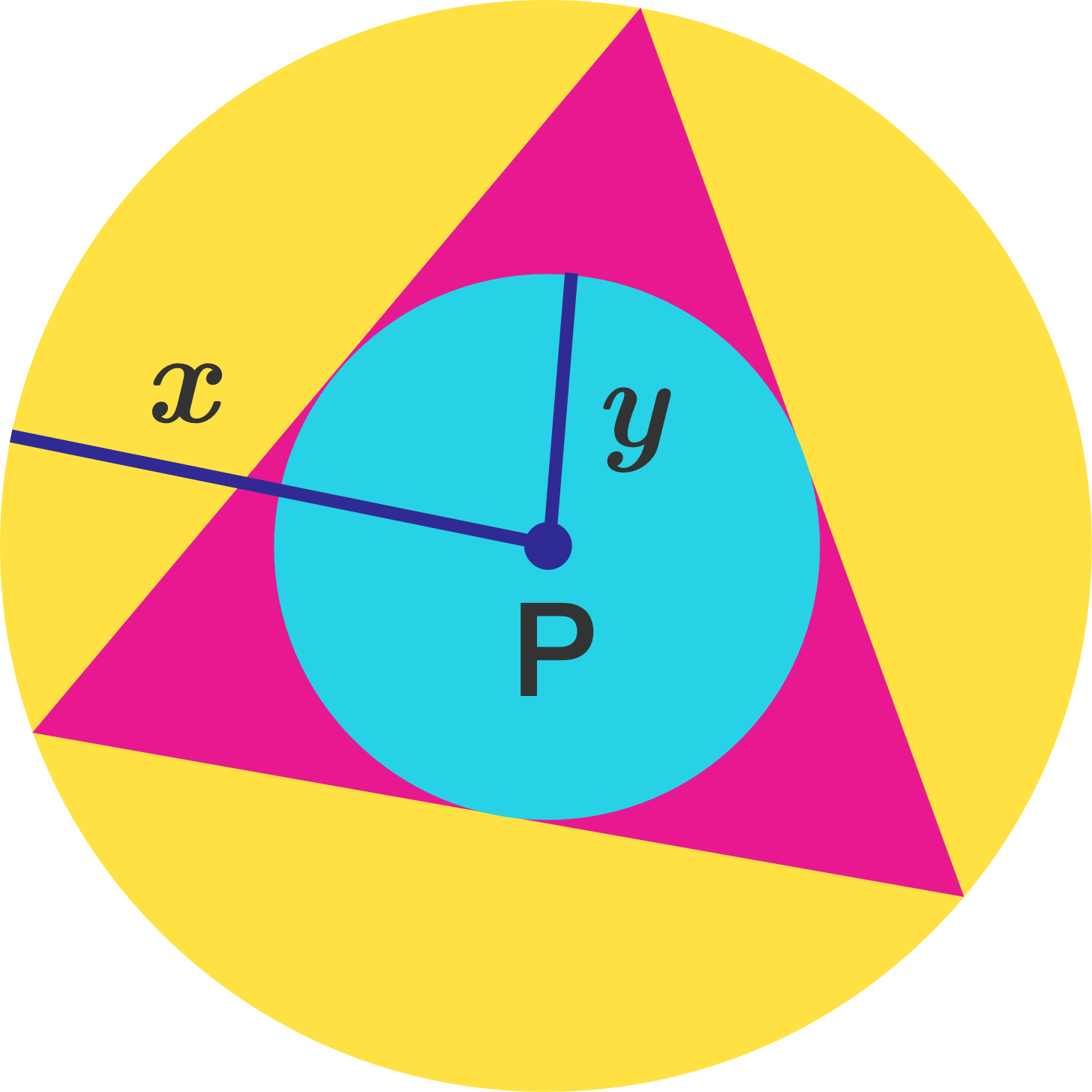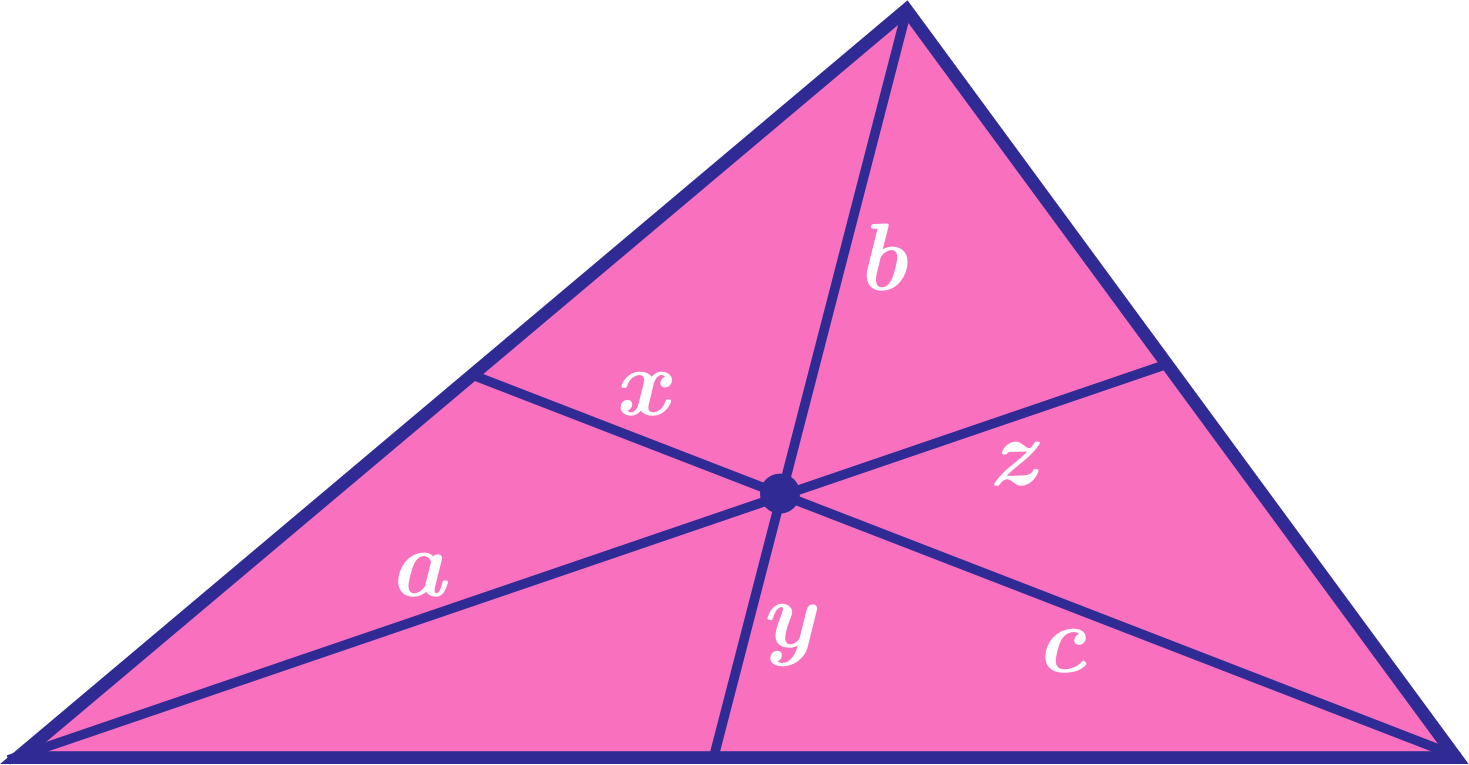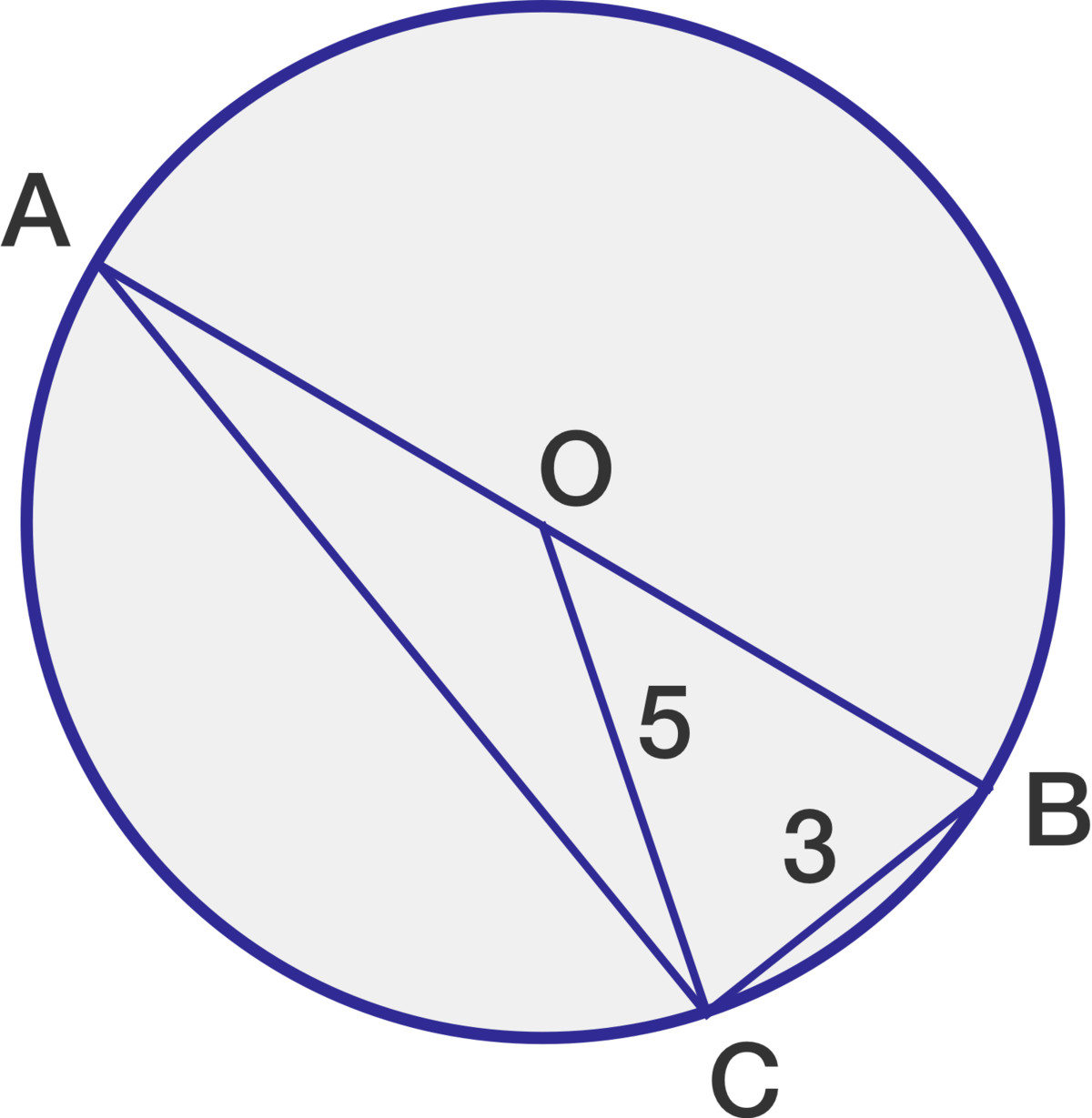Everyday Math

# Geometry Shortcuts

True or false?

A circle can be inscribed in a kite.What is the value of $y$ in the diagram shown? (HINT: Think ratios)Given the two circles with center P and the equilateral triangle shown, if the value of $x,$ the radius of the larger circle, is 8, what is the value of $y,$ the radius of the smaller circle?In the triangle shown, the three line segments meet at the centroid of the triangle. How many times greater is the product $abc$ than the product $xyz?$ (HINT: The centroid of a triangle trisects each line segment)Given that AB is the diameter of the circle shown with center at $O,$ what is the approximate value of AC?

×